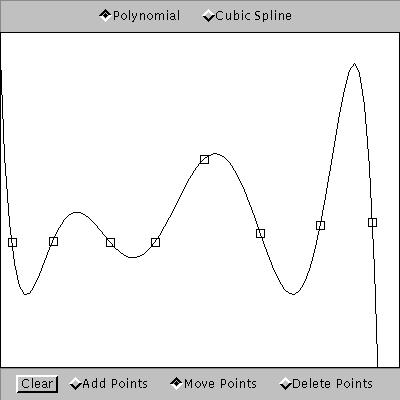# Interpolation with Polynomials and Splines

This uses Java, so you need to have a Java plugin installed and enabled in your browser.

In the applet below you can choose a number of points and see the polynomial and the natural cubic spline passing through the given points.

Your browser cannot use Java, or you deactivated Java in your browser. Here is a static image of the applet:For n given points there exists a unique polynomial of degree n-1 or less which passes through these points.

A cubic spline is a piecewise cubic polynomial such that the function, its derivative and its second derivative are continuous at the interpolation nodes. The natural cubic spline has zero second derivatives at the endpoints. It is the smoothest of all possible interpolating curves in the sense that it minimizes the integral of the square of the second derivative.

Try to put about eight points in a straight line. Then move one of the points in the middle up and down. You will see that the interpolating polynomial will change drastically even far away from the perturbed node. For the cubic spline, however, the changes rapidly decay away from the perturbed node.

(Part of the code was stolen from Michael Heinrichs' Curve Applet. The source code is available at www.math.umd.edu/~tvp/Interp2_code.java)

The standard reference for splines is

A Practical Guide to Splines by Carl De Boor, Springer 2001
which contains Fortran code for all algorithms.